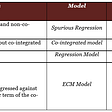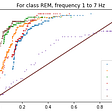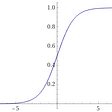# Learning Hidden Markov Models with simple examples and Matlab [part 1]

Once I’d finished doing the exercises I’ll show in this article, I realized they helped me a lot in understanding Hidden Markov Models (HMMs). That’s why I thought this might be a helpful article for someone trying to grasp the basic concepts of HMMs in a practical way. The information will be delivered in 2 approaches — a pen-and-paper one and a coding one.

Prerequisites for the coding part:

*If you’re a student, there’s a high probability that you can get Matlab with a student’s license, or maybe your university provides Matlab licenses. If not, at the time of writing, there’s a 30-day test version and you can use that to try out the coding part.

So, whenever I start learning something new, my go-to method is to create a basic foundation that I fully comprehend and then start building on top of it to understand more complex concepts.

If you’re not sure if you’ve got this “foundation” regarding HMMs, I’d recommend going through mathematicalmonk’s YouTube series on HMMs. It’s by far much better than any kind of summary I’d be able to write here. Now, assuming the main ideas of HMMs are familiar to you, let’s get to the first problem that would hopefully help you understand HMMs better.

## HMM the old-fashioned way (pen-and-paper part)

Consider an HMM with the following properties:

• Hidden state set Q = {1,2,3}
• Observable state set V = {a, b}
• Parameter λ = (π, A, B)

where matrices A (transition matrix), B (emission matrix), π (initial distribution) are defined as follows:

We concern ourselves with a small time step of T = 3 with the observed sequence being O = (a, b, a).

Given this information, we’ll need to:

1. Use the Forward algorithm to calculate P(O|λ). So, what is the probability that such a sequence “a → b → a” actually occurs?
2. Find the optimal hidden state sequence I* = (i₁*, i₂*, i₃*). Meaning, which are the 3 hidden states with the highest possibility of being behind the sequence “a →b → a”?

Solution to part 1:

1. The approach here is to go step by step and calculate what’s the probability of observing the current state given the previously observed states at each time step. So, for the first state that would mean:
• The (initial distribution probability) x (probability of observing state a” given the previous states) = P(state 0) x P(a|state Zero).

Continuing, we go to the second state which follows the definition of the Forward algorithm. However, unlike the calculation for the first state, here we have to consider the result from the previous state (first state) and include it in the calculations. This results in a sum over the product of probabilities of observing a previous state (α₁ from the calculation in the upper picture) multiplied by the probabilities of the current state given the previous ones. If you feel lost in this explanation (I tried my best, but I wouldn’t blame you), just compare the vectors in the formula to the matrix columns of B and matrix rows of A and you’ll get a feel for what exactly’s going on.

Now, if you’ve understood what’s going on so far, you might guess that the process of applying the Forward algorithm would be the exact same for all future states, you’ll just have different numbers to plug in. And you’re right! So, try and compute the result for the third state before looking at my solution.

We’re almost done. We’ve gone one by one through each observed state at the 3 steps and now there’s this vector we’ve calculated after applying the Forward algorithm 3 times. But what is it, really?

It’s a representation of the probabilities of having observed the sequence “a → b → a” and ending up in:

• Hidden state 1 with a chance of 4.187%
• Hidden state 2 with a chance of 3.5512%
• Hidden state 3 with a chance of 5.2836%

Now, if you sum up all three percentages you’ll get the total probability of actually observing “a → b → a” regardless of which hidden state you end up in because that’s of no interest so far.

There you have it, the probability of actually observing O = (a, b, a) is approximately 13%.

Hopefully, this article was concise and straightforward, but most of all, I hope it was helpful. I’ll see that the solution to the second part is online within the next couple of days, and after that, I’ll write the last article that discusses an HMM problem solved using Matlab and its great features.

Comments, corrections, and other remarks are always appreciated.

Sources:
Thumbnail picture: https://devopedia.org/images/article/214/3976.1567701977.png

--

--

--

Electrical engineer turned software (or I’d like to think so). Trying to learn + share new topics. Past internship blog posts: https://hack.bg/author/aleksandar

Love podcasts or audiobooks? Learn on the go with our new app.

## Bayes’ Theorem and Shark Attacks## How can we simply approximate the fuure?## Big Numbers Aren’t Working Anymore## Error Correction Model (ECM): An Intuitive Guide## Strange Attractor## Algebra and Group Theory## CAT Tricks By A 99.97%iler — Series 1 On Quant## Tips And Tricks For How To Be Good At Math For StudentsElectrical engineer turned software (or I’d like to think so). Trying to learn + share new topics. Past internship blog posts: https://hack.bg/author/aleksandar

## Machine Learning an introduction — Thoughts on programming## Treasure Maze Reinforced Learning## Proving for yourself, through Deep Learning, that you dream in REM!## Manufacturing polynomials using a sigmoid neural network